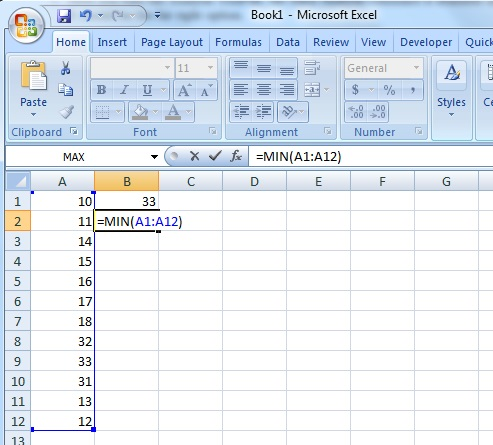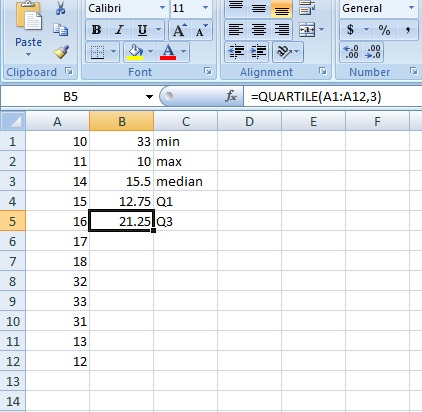# 5 Number Summary in Excel: Easy Steps with Video

Share on

Probability and Statistics > Basic Statistics>

5 Number Summary in Excel
Watch the video for the steps:

## How to find a 5 number summary in Excel

A five number summary is a way to describe data. It is made up of five statistics:

• The median (the middle).
• The maximum value.
• The minimum value.
• Q1, the first quartile.
• Q3, the third quartile.

The first quartile marks the line at the 25th percentile of data and the third quartile marks the link at the 75th percentile. The data that lies between these two points is called the interquartile range.
Excel doesn’t have a single command to calculate a five number summary, but you can find a 5 number summary in Excel by using basic functions to calculate the median, max, minimum, Q1 and Q3.

## 5 Number Summary in Excel: Steps

Example question: Calculate the five number summary in Excel for the following data set: 10, 11, 14, 15, 16, 17, 18,, 32, 33, 31, 13, 12.

Step 1: Type your data into a single column in Excel. For this example question, type the values into cells A1 through A12.

Step 2: Click an empty cell, then type “MAX(A1:A12)” (without parentheses). A1 is the first cell your data is in, A12 is the last. The two values are separated by a colon. Press “Enter” to find the max: 33.

Step 3: Repeat Step 2 for the minimum: “MIN(A1:A12)”.Step 4: Repeat Step 2 for the median: “MEDIAN(A1:A12)”.

Step 5: Repeat Step 2 for the first quartile: “QUARTILE(A1:12,1)”. The “1” after A1:a12 lets Excel know you want the first quartile.

Step 6: Repeat Step 2 for the third quartile: “QUARTILE(A1:12,3)”. The “3” after A1:a12 lets Excel know you want the third quartile.Your five number summary is in B1:B5.

Related articles:
How to Find a Five Number Summary

Check out our YouTube channel for more stats help and tips!

CITE THIS AS:
Stephanie Glen. "5 Number Summary in Excel: Easy Steps with Video" From StatisticsHowTo.com: Elementary Statistics for the rest of us! https://www.statisticshowto.com/find-5-number-summary-in-excel/
---------------------------------------------------------------------------Need help with a homework or test question? With Chegg Study, you can get step-by-step solutions to your questions from an expert in the field. Your first 30 minutes with a Chegg tutor is free!